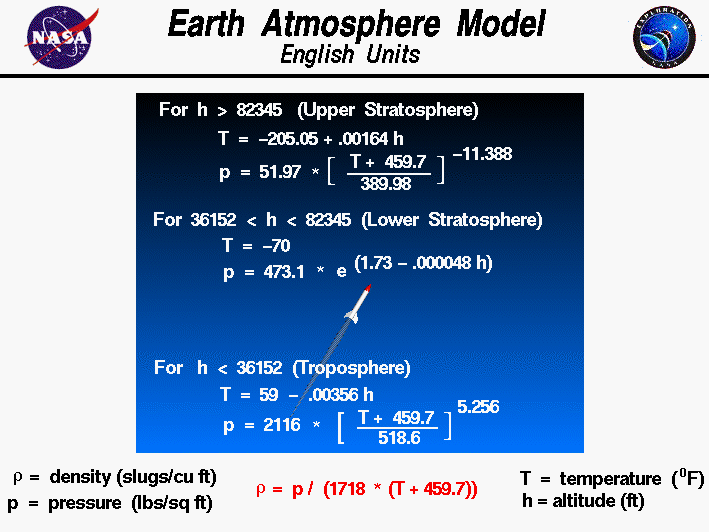+ Text Only Site
+ Non-Flash Version
+ Contact GlennThe Earth's atmosphere is an extremely thin sheet of air extending from the surface of the Earth to the edge of space, about 60 miles above the surface of the Earth. If the Earth were the size of a basketball, a tightly held pillowcase would represent the thickness of the atmosphere. Gravity holds the atmosphere to the Earth's surface. Within the atmosphere, very complex chemical, thermodynamic, and fluid dynamics effects occur. The atmosphere is not uniform; fluid properties are constantly changing with time and place. We call this change the weather. Variations in air properties extend upward from the surface of the Earth. The sun heats the surface of the Earth, and some of this heat goes into warming the air near the surface. The heated air is then diffused or convected up through the atmosphere. Thus the air temperature is highest near the surface and decreases as altitude increases. The speed of sound depends on the temperature and also decreases with increasing altitude. The pressure of the air can be related to the weight of the air over a given location. As we increase altitude through the atmosphere, there is some air below us and some air above us. But there is always less air above us than was present at a lower altitude. Therefore, air pressure decreases as we increase altitude. The air density depends on both the temperature and the pressure through the equation of state and also decreases with increasing altitude. Aerodynamic forces directly depend on the air density. To help rocket designers, it is useful to define a standard atmosphere model of the variation of properties through the atmosphere. There are actually several different models available--a standard or average day, a hot day, a cold day, and a tropical day. The models are updated every few years to include the latest atmospheric data. The model was developed from atmospheric measurements that were averaged and curve fit to produce the given equations. The model assumes that the pressure and temperature change only with altitude. The particular model shown here was developed in the early sixties, and the curve fits are given for English units. Curve fits are also available in metric units. The model has three zones with separate curve fits for the troposphere, the lower stratosphere, and the upper stratosphere. The troposphere runs from the surface of the Earth to 36,152 feet. In the troposphere, the temperature decreases linearly and the pressure decreases exponentially. The rate of temperature decrease is called the lapse rate. For the temperature T and the pressure p, the English units curve fits for the troposphere are: T = 59 - .00356 * h p = 2116 * [(T + 459.7)/ 518.6]^5.256 where the temperature is given in Fahrenheit degrees, the pressure in pounds/square feet, and h is the altitude in feet. The lower stratosphere runs from 36,152 feet to 82,345 feet. In the lower stratosphere the temperature is constant and the pressure decreases exponentially. The English units curve fits for the lower stratosphere are: T = -70 p = 473.1 * exp(1.73 - .000048 * h) The upper stratosphere model is used for altitudes above 82,345 feet. In the upper stratosphere the temperature increases slightly and the pressure decreases exponentially. The English units curve fits for the upper stratosphere are: T = -205.05 + .00164 * h p = 51.97 * [(T + 459.7)/ 389.98]^-11.388 In each zone the density r is derived from the equation of state. r = p / [1718 * (T + 459.7)] This is the atmosphere model used in the RocketModeler II simulator. An interactive simulation for the atmosphere model is also available. With the applet, you can change altitude and see the effects on pressure and temperature. Guided ToursOur Neighbors in Space:Standard Atmosphere Model:Function:Atmosphere Simulator:Activities: Related Sites: Rocket Index Rocket Home Beginner's Guide Home+ Inspector General Hotline + Equal Employment Opportunity Data Posted Pursuant to the No Fear Act + Budgets, Strategic Plans and Accountability Reports + Freedom of Information Act + The President's Management Agenda + NASA Privacy Statement, Disclaimer, and Accessibility CertificationEditor: Tom Benson NASA Official: Tom Benson Last Updated: May 13 2021 + Contact Glenn